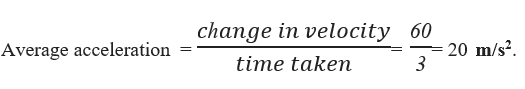# Average Acceleration Formula

## Average Acceleration Formulas - Definition & Examples

Acceleration for an object is defined as the rate of change of velocity.
Average acceleration for an interval is defined as the change in velocity for that interval per time

So, mathematically $acceleration\left( a \right) = \frac{{\Delta v}}{{\Delta t}}$
If vi is the initial velocity and vf is the final velocity, and t is the time taken for the velocity to change from vi to vf , then$a = \frac{{vf - vi}}{t}$

SI unit of average acceleration is m/s2.

Example:
If an object accelerates from 20 m/s to 80 m/s in 3 seconds, Find the average acceleration for the object.
Ans:Question:

If an object accelerates from rest with an acceleration of 3 m/s2 for 5 seconds, Find the final velocity.